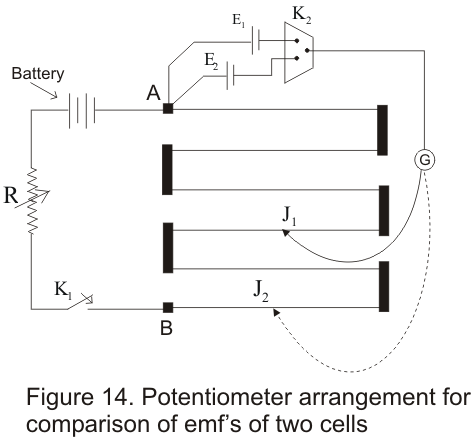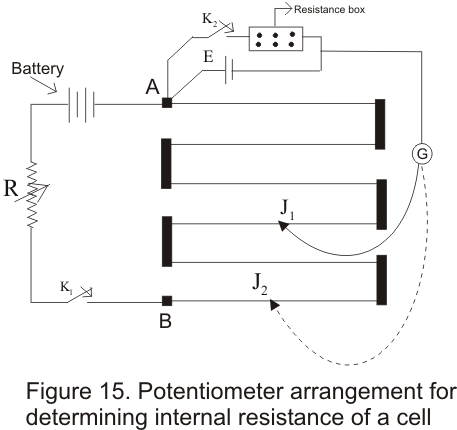# Application of potentiometer

## (A) Comparison of EMF's of two cells using potentiometer

• Consider the circuit arrangement of potentiometer given below used for comparison of emf's of two cells• Positive terminals of two cells of emf's E1 and E2( whose emf are to be compared ) are connected to the terminals A and negative terminals are connected to jockey through a two way key K2 and a galvanometer
• Now first key K1 is closed to establish a potential difference between the terminals A and B then by closing key Ksub>2 introduce cell of EMF E1 in the circuit and null point junction J1 is dtermined with the help of jockey.If the null point on wire is at length
l1 from A then
E1=Kl1
Where K -> Potential gradient along the length of wire
• Similarly cell having emf E2 is introduced in the circuit and again null point J2 is determined .If length of this null point from
A is l2 then
E2=Kl2
Therefore
E1/E2=l1/l2
This simple relation allows us to find the ratio of E1/E2
• if the EMF of one cell is known then the EMF of other cell can be known easily

## (B) Determination of internal resistance of the cell

• Potentiometer can also be used to determine the internal resistance of a cell• For this a cell whose internal resistance is to be determined is connected to terminal A of the potentiometer across a resistance box through a key K2
• First close the key K1 and obtain the null point .Let l1 be the length of this null point from terminal A then
E=Kl1
• When key K2 is closed ,the cell sends current through resistance Box (R).If E2 is the terminal
Potential difference and null point is obtained at length l2(AJ2) then
V=Kl2
Thus
E/V=l1/l2
But E=I(R+ r) and V=IR
This gives
E/V=(r+R)/R
So (r+R)/R=l1/l2
giving
r=R(l1/l2-1)
• Using above equation we can find internal resistance of any given cell